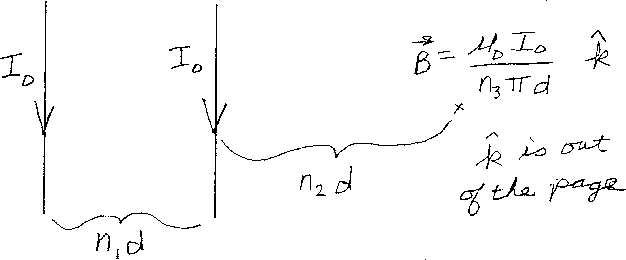Problem C2: In the figure above, there are two infinitely long thin wires. Each wire carries the same current I0 downward. The wires are separated by a distance n1d. What is the magnetic field at a location which is a distance n2d to the right of wire on the right? That is, if the magnetic field at this location equals μ0 I0/(n3 π d) k, where k points out of the page. What is n3?n1 = n2 = Input n3:
If you are currently in my class, you can record your grade by entering your name and student ID number (without the leading zeros) below and clicking on "record grade".
 First Name = Last Name = ID = Problem: